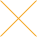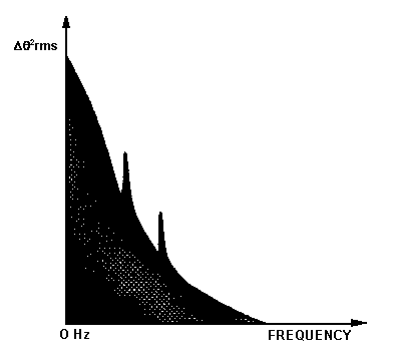Our website uses cookies. By continuing to use this website, you consent to cookies being used.
English
English

# Characterizing and Minimizing VCO Phase Noise

## Characterizing Phase Noise

The term phase noise is widely used for describing short term random frequency fluctuations of a signal. Frequency stability is a measure of the degree to which an oscillator maintains the same value of frequency over a given time. This may be specified in a number of ways. Three commonly used terms for describing frequency stability are used here. An ideal sine wave oscillator may be described by:

V(t) = Vo Sin2Pft

Here, Vo is the nominal amplitude of the signal, and f is the nominal frequency of oscillation. The instantaneous output of an oscillator may be represented by:

V(t) = Vo {1 + A(t)} Sin {2P ft + q (t)}

where A(t) and q(t) represent the amplitude and phase fluctuations of the signal respectively. The phase term may be random or discrete and could be seen on the spectrum analyzer. As shown in Figure 1 there are two types of fluctuating phase terms. The first, the discrete signals called spurious, appear as distinct components in the spectral density plot. The second term, random in nature, appears as random phase fluctuations and is commonly called phase noise.

The source of phase noise in an oscillator is due to thermal and flicker or 1/f noise. Most oscillators operate in saturation. The AM noise component is usually 20dB lower than the phase noise component. In the discussion that follows, we will assume that A(t)<<1.

Many methods are used to characterize phase noise of an oscillator. Essentially, all methods measure the frequency or phase deviation of the source under test in either frequency or time domain. Since frequency and phase are related to each other, all these terms are also related. One of the most common fundamental descriptions of phase noise is the one sided spectral density of phase fluctuations per unit bandwidth. The term spectral density describes the energy distributions as a continuous function, expressed in units of energy per unit bandwidth. The phase noise of an oscillator is best described in the frequency domain where the spectral density is characterized by measuring the noise sidebands on either side of the output signal center frequency. Single sideband phase noise is specified in dBc/Hz at a given frequency offset from the carrier. The frequency domain information about phase or frequency is contained in the power spectral density SDq(f) of the phase or in the power spectral density SDf(f) of the frequency. Here, f refers to the modulation frequency or offset frequency associated with the noise-like variations in q(t).

Peak phase modulation Dq and peak frequency modulation Df are related as follows:

In terms of rms values, we have:

The one sided spectral distribution of the phase fluctuations per Hz bandwidth is SDq(f):

Here, BW is the bandwidth of Dqrms measurement. The units of SDq(f) are radian2 Hz-1 bandwidth or dB relative to 1 rad2 Hz-1 bandwidth. The term S= (f) is often referred to as the spectral density and describes the energy distribution as a continuous function, expressed in units of energy per Hz bandwidth. This is illustrated in Figure 2.Figure 2: One sided representation of phase noise spectral density of signal

Similarly, one sided spectral distribution of the frequency fluctuations per Hz bandwidth is SDf(f) where:

SDf = (Dfrms)2 / BW

Here, BW is the bandwidth of Dfrms measurement. The units of SDf(f) are (rad sec-1)2 HZ-1 bandwidth. It is also common to characterize the noise performance of a signal as the ratio of the sideband power associated with phase fluctuations to the carrier power level. If the measure is denoted by Sc

Sc(f) = (Power density in one sideband per Hz bandwidth at an offset frequency f away from the carrier) / (Total signal power) For small phase fluctuations, we can write:

Here, b is the modulation index by analogy to modulation theory.

Sc(f) = 1/2 (SDq(f))

SCBfl is often expressed in decibels relative to the carrier per Hz c/Hz) and is related to the power spectrum observed on a spectrum analyzer. The National Bureau of Standards defines Single Side Band Phase Noise as the ratio of power in one phase modulation sideband per Hertz bandwidth, at an offset f Hertz away from the carrier, to the total signal power. Here, f is the offset frequency from the carrier.

where Ps is the carrier power and Pssb is the sideband power in one Hz bandwidth at an offset frequency of f from the center. This is illustrated in Figure 3.

The Single Sideband Phase Noise is usually given logarithmically, that is:

Sc(f) in dB = 10 x log[Sc(f)]

This is shown in Figure 4 as a spectral density plot of the phase modulation sidebands in the frequency domain. It is expressed in dB relative to the carrier per Hz bandwidth.

The phase noise generated by a VCO is determined by:

1. Q factor of the resonator
2. Q of the varactor diode
3. the active device used for the oscillating transistor
4. power supply noise
5. external tuning voltage supply noise

The noise contribution made by (d) and (e) can be minimized by careful choice of the power supplies (see Application Note 3). The phase noise of the VCO is therefore determined primarily by the overall Q of the circuit. In order to design a circuit with high Q, the tuning bandwidth must be made small. Therefore a VCO designed for low phase noise performance will have a smaller tuning range.

## Ways to minimize noise

The following steps are recommended for obtaining the best overall performance from Mini-Circuits VCO's.

1. Power Supply (Vcc) and tuning voltage (Vtune) returns must be connected to the printed circuit board ground plane. VCO ground plane must be the same as that of the printed circuit board and therefore all VCO ground pins must be soldered direct to the printed circuit board ground plane.
2. Adequate RF grounding is required. Several chip decoupling capacitors must be provided between the Vcc supply and ground.
3. Good, low noise power supplies must be used. Ideally, DC batteries for both supply (VCC) and tuning (Vtune) voltages will provide the best overall performance.
4. Output must be correctly terminated with a good load impedance. It is also a good practice to use a resistive pad between the VCO and the external load.
5. Connections to the tuning port must be as short as possible and must be well screened, shielded, and decoupled to prevent the VCO from being modulated by external noise sources. A low noise power supply must be used for tuning voltage (Vtune) Supply.